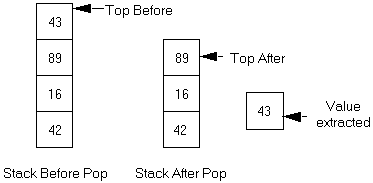Computer Science - C++ Lesson 40:  Stacks

OBJECTIVES:

The student will use stacks to solve a non-recursive inorder tree traversal.

INTRODUCTION:

When studying recursion you were introduced to the concept of a stack.  A stack is a linear data structure with well defined insertion and deletion routines.  The stack abstraction has been solved for you in the apstack class.  After covering the member functions available in the apstack class the lab exercise will use stacks to solve a non-recursive inorder tree traversal problem.

The key topics for this lesson are:

A.  The Stack Abstract Data Type            B.   Implementation Strategies for a Stack Type

VOCABULARY:

 PUSH STACK POP TOP

DISCUSSION:

A.  The Stack Abstract Data Type

1.   The stack object is a linear data structure, with each node or cell holding the same data type.

2.   All additions to and deletions from a stack occur at the top of the stack.  The last item pushed onto the stack will be the first item removed.  A stack is sometimes referred to as a LIFO structure, which stands for Last-In, First-Out.

3.   Two of the more important stack operations involve pushing data onto a stack and popping data off the stack.

4.   The push operation will look like this:

Push Operation5.   The pop operation will look like this:

Pop Operation:See Handout H.A.40.1, apstack. 6.   Here is a summary of the stack operations supported by the apstack class.  See Handout H.A.40.1, apstack for the details.

Constructors

apstack();                                      constructs an empty stack

apstack (const apstack &s);      copy constructor

~apstack ();                                  destructor

Assignment

const apstack&  operator= (const apstack& rhs);

Accessors

const itemType &  top()  const; returns top element with no pop

bool  isEmpty() const;                  return true if stack is empty, else false

int  length() const;                       returns length of stack

Modifiers

void push (const itemType & item);          push item onto top of stack

void pop ();                                                    pop top element

void pop (itemType& item);                        combines pop and top

void makeEmpty();                                      empties stack

7.   The distinction between top and pop follows:  the top operation simply returns the top value in the stack while the pop operation removes the top value.  Notice that the pop operation has been overloaded to either remove the top element, or to remove and return the top element.

8.   Here is a short program illustrating usage of the apstack class.

// Example program of using apstack class

#include <iostream.h>

#include <apstack.h>

main()

{

apstack<int> stack;

int value;

for (int k=1; k<=5; k++)

stack.push(k);

while (!(stack.isEmpty()))

{

stack.pop(value);

cout << value << "  ";

}

return 0;

}

B.   Implementation Strategies for a Stack Type

1.   The data structure used in the apstack class is an apvector.  This allows for resizing of the stack as needed to make it larger.  However the author of the apstack class did not resize the apvector smaller as the stack was popped.  A vector is an appropriate data structure to use to implement a stack.

2.   Another approach would be to use a linked list which would support true dynamic resizing.  As you push data onto the stack another node is added to the appropriate end of the linked list.  When data is popped from the stack, the linked list would be reduced in size.

3.   A future lesson will teach you how to write templated classes.  You will change the implementation of the apstack class while leaving the interface stable.

SUMMARY/REVIEW:         The stack ADT is what makes recursive algorithms possible.  In the lab exercise you will gain a better understanding of the recursive inorder function used to traverse a binary tree.

ASSIGNMENT:                    Lab Exercise L.A.40.1, Inorder

Background:

Any recursive algorithm can be reduced to a linear sequence of events.  It will be longer and more difficult to follow, but recursive solutions can be rewritten as iterative solutions if a stack is available for use.  In this lab exercise, you will implement a non-recursive inorder function now that we have the apstack class to support stack operations.  After completing the lab, you should have a greater appreciation for recursion and what it will accomplish for you.

You will work with the same binary tree code as implemented in Lessons 36-38.  A non-recursive inorder function is summarized in pseudocode form below.

void  inorder (treePtr  root)

{

declare a stack of treePtr, initialized as empty

declare temp as a treePtr

start temp = root

do

while moving temp as far left as possible, push tree pointers onto the stack

if the stack is not empty

reposition temp by popping the stack

print the contents of temp-> data

move temp one node to the right

while (the stack is not empty) or (temp != NULL)

Assignment:

1.   Starting with an old binary tree lab, keep the code needed to read a data file (File20.txt) and build the binary tree.

2.   Add #include <apstack.h> to the top of your program to access the stack ADT.

3.   Solve the code for the non-recursive inorder function.  Use (file20.txt) to test your program.